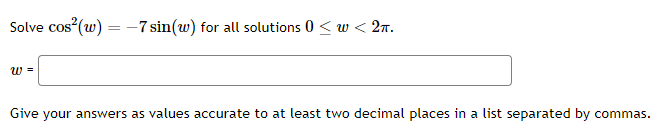# (Solved): Solve cos2(w)=7sin(w) for all solutions 0w<2 w= Give your answers as values accurate to ...Solve for all solutions Give your answers as values accurate to at least two decimal places in a list separated by commas.

We have an Answer from Expert

To solve the equation    for all solutions in the interval    it can use trigonometric identities to simplify and solve the equation.

Use the trigonometric identity to replace    in the equation:
It implies that

Rearrange the equation to form a quadratic equation:

The trigonometric identity    is used

We have an Answer from Expert